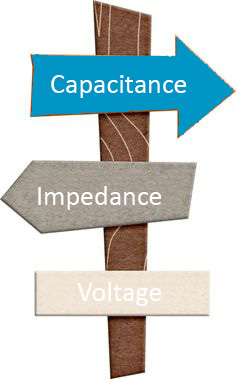CONSIDER THIS: Electrical Requirements

Posted on Wednesday, January 6, 2016 by Kimberly Versaw

When choosing a wire or cable, one of the most important factors to take into consideration is the electrical requirements.  Below are the items to consider when understanding the appropriate electrical requirements.

Ampacity (Current Carrying Capacity): The amount of current (electrical flow) a conductor can carry. The larger the wire size, the greater the amount of current. Current is expressed in amperes (Amp).

Memory trick: Think of a garden hose. It is the amount of water that the hose can carry. The larger the hose, the more water it can carry.

Capacitance: The storage of electrically separated charges between two conductor surfaces having different potentials. The value depends upon the surface area of the conductors and the distance between them. Capacitance is expressed in Pico farad per foot (pF/ft).

Memory trick: Think of a storage tank. The larger the tank, the more room for storage and the smaller the tank, the less room for storage.
• Direct Capacitance: Measured directly between conductors.
• Mutual Capacitance: Measured between two conductors with all other conductors and shield tied to ground.
• Ground Capacitance: Capacitance between one conductor and all other conductors tied together and to the grounded shield.

Inductance: The property of a circuit or circuit element that opposes a change in current flow, causing current changes to lag behind voltage changes. This is expressed in micro henries per foot (μH/Ft).

Memory trick: Think of a river. When there is no blockage the water flows without any problem; however, if a beaver has built a dam then the water isn’t able to flow past it, it starts backing up and therefore it is more difficult for the water to flow.

Impedance: The total opposition (reactance, X & resistance, R) that a circuit offers to the flow of alternating current (AC) at any given frequency. Impedance is expressed in Ohms (Ω). Impedance is not length related.

• Characteristic Impedance: The ratio of voltage to current at each point along a transmission line on which there are no standing waves.

Resistance: A measurement of the difficulty in moving electrical current through a medium when voltage is applied. Resistance is expressed in Ohms (Ω).

Memory trick: Think of a highway. When there is a traffic jam it is more difficult to get through than when there is no traffic.
• In DC circuits, resistance is the opposition that a material offers to the current flow.
• In AC circuits, resistance is a component of impedance, which is normally higher than the actual measured DC resistance value.

Velocity of Propagation (Vp): The speed a signal travels down a length of cable as compared to the speed in free air and is directly dependent upon the dielectric material properties. Vp is the reciprocal of the square root (√) of the dielectric constant (ε) of the insulation material (1/√ε) and is expressed as a percentage (%).

Memory trick: Think of a faucet. If you open your bath tub faucet all the way the tub will fill quicker than if you just barely turn the faucet on.

Voltage (Volt): A measurement of electrical pressure along a conductor. The voltage rating depends primarily on the insulation thickness. The thicker the insulation, the higher the voltage rating.

• 0.010” nominal insulation wall = 600 volts (Military Specifications)
• 0.016” nominal insulation wall = 1000 volts (Military Specifications)
• 0.016” nominal insulation wall = 300 volts (UL)
• 0.032” nominal insulation wall = 600 volts (UL)

Share:Archive